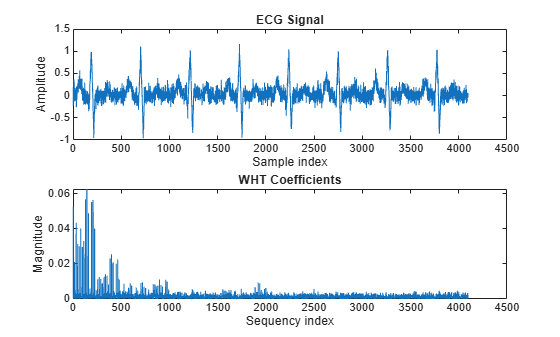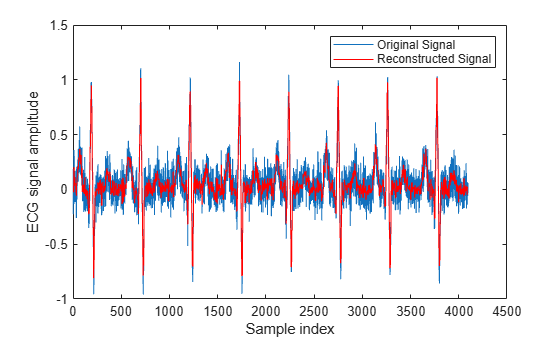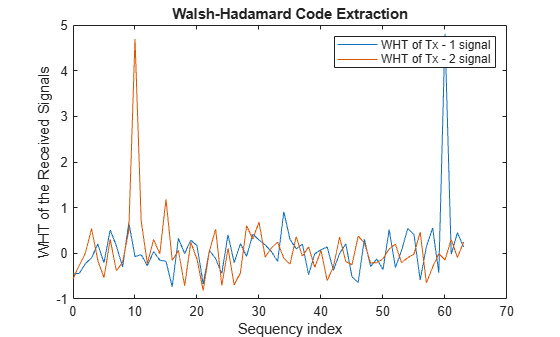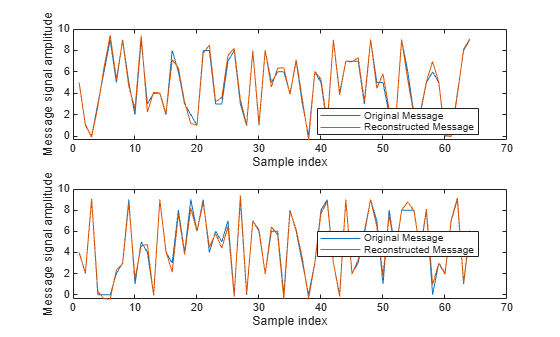### Introduction

This example shows how to use the Walsh-Hadamard transform (WHT) and some of its properties by showcasing two applications: communications using spread spectrum and processing of ECG signals.

WHTs are used in many different applications, such as power spectrum analysis, filtering, processing speech and medical signals, multiplexing and coding in communications, characterizing non-linear signals, solving non-linear differential equations, and logical design and analysis.

The WHT is a suboptimal, non-sinusoidal, orthogonal transformation that decomposes a signal into a set of orthogonal, rectangular waveforms called Walsh functions. The transformation has no multipliers and is real because the amplitude of Walsh (or Hadamard) functions has only two values, +1 or -1.

Walsh functions are rectangular or square waveforms with values of -1 or +1. An important characteristic of Walsh functions is sequency which is determined from the number of zero-crossings per unit time interval. Every Walsh function has a unique sequency value.

Walsh functions can be generated in many ways (see ). Here we use the `hadamard` function in MATLAB® to generate Walsh functions. Length eight Walsh functions are generated as follows.

```N = 8; % Length of Walsh (Hadamard) functions hadamardMatrix = hadamard(N)```
```hadamardMatrix = 8×8 1 1 1 1 1 1 1 1 1 -1 1 -1 1 -1 1 -1 1 1 -1 -1 1 1 -1 -1 1 -1 -1 1 1 -1 -1 1 1 1 1 1 -1 -1 -1 -1 1 -1 1 -1 -1 1 -1 1 1 1 -1 -1 -1 -1 1 1 1 -1 -1 1 -1 1 1 -1 ```

The rows (or columns) of the symmetric `hadamardMatrix` contain the Walsh functions. The Walsh functions in the matrix are not arranged in increasing order of their sequencies or number of zero-crossings (i.e. 'sequency order') but are arranged in 'Hadamard order'. The Walsh matrix, which contains the Walsh functions along the rows or columns in the increasing order of their sequencies is obtained by changing the index of the `hadamardMatrix` as follows.

```HadIdx = 0:N-1; % Hadamard index M = log2(N)+1; % Number of bits to represent the index```

Each column of the sequency index (in binary format) is given by the modulo-2 addition of columns of the bit-reversed Hadamard index (in binary format).

```binHadIdx = fliplr(dec2bin(HadIdx,M))-'0'; % Bit reversing of the binary index binSeqIdx = zeros(N,M-1); % Pre-allocate memory for k = M:-1:2 % Binary sequency index binSeqIdx(:,k) = xor(binHadIdx(:,k),binHadIdx(:,k-1)); end SeqIdx = binSeqIdx*pow2((M-1:-1:0)'); % Binary to integer sequency index walshMatrix = hadamardMatrix(SeqIdx+1,:) % 1-based indexing```
```walshMatrix = 8×8 1 1 1 1 1 1 1 1 1 1 1 1 -1 -1 -1 -1 1 1 -1 -1 -1 -1 1 1 1 1 -1 -1 1 1 -1 -1 1 -1 -1 1 1 -1 -1 1 1 -1 -1 1 -1 1 1 -1 1 -1 1 -1 -1 1 -1 1 1 -1 1 -1 1 -1 1 -1 ```

The forward and inverse Walsh transform pair for a signal x(t) of length N are

`${y}_{n}=\frac{1}{N}\sum _{i=0}^{N-1}{x}_{i}WAL\left(n,i\right),n=1,2,\dots ,N-1$`

`${x}_{i}=\sum _{n=0}^{N-1}{y}_{n}WAL\left(n,i\right),i=1,2,\dots ,N-1$`

Fast algorithms, similar to the Cooley-Tukey algorithm, have been developed to implement the Walsh-Hadamard transform with complexity $\mathit{O}\left(\mathit{N}\text{\hspace{0.17em}}\mathrm{log}\text{\hspace{0.17em}}\mathit{N}\right)$ (see  and ). Since the Walsh matrix is symmetric, both the forward and inverse transformations are identical operations except for the scaling factor of 1/N. The functions `fwht` and `ifwht` implement the forward and the inverse WHT respectively.

Example 1 Perform WHT on the Walsh matrix. The expected result is an identity matrix because the rows (or columns) of the symmetric Walsh matrix contain the Walsh functions.

`y1 = fwht(walshMatrix) % Fast Walsh-Hadamard transform`
```y1 = 8×8 1 0 0 0 0 0 0 0 0 1 0 0 0 0 0 0 0 0 1 0 0 0 0 0 0 0 0 1 0 0 0 0 0 0 0 0 1 0 0 0 0 0 0 0 0 1 0 0 0 0 0 0 0 0 1 0 0 0 0 0 0 0 0 1 ```

Example 2 Construct a discontinuous signal by scaling and adding arbitrary columns of the Hadamard matrix. This signal is formed using weighted Walsh functions, so the WHT should return non-zero values equal to the weights at the respective sequency indices. While evaluating the WHT, the `ordering` is specified as `'hadamard'`, because a Hadamard matrix (instead of the Walsh matrix) is used to obtain the Walsh functions.

```N = 8; H = hadamard(N); % Hadamard matrix % Construct a signal by adding a few weighted Walsh functions x = 8.*H(1,:) + 12.*H(3,:) + 18.*H(5,:) + 10.*H(8,:); y = fwht(x,N,'hadamard')```
```y = 1×8 8 0 12 0 18 0 0 10 ```

WHT is a reversible transform and the original signal can be recovered perfectly using the inverse transform. The norm between the original signal and the signal obtained from inverse transformation equals zero, indicating perfect reconstruction.

```xHat = ifwht(y,N,'hadamard'); norm(x-xHat)```
```ans = 0 ```

The Walsh-Hadamard transform involves expansion using a set of rectangular waveforms, so it is useful in applications involving discontinuous signals that can be readily expressed in terms of Walsh functions. Below are two applications of Walsh-Hadamard transforms.

### Walsh-Transform Applications

ECG signal processing Often, it is necessary to record electro-cardiogram (ECG) signals of patients at different instants of time. This results in a large amount of data, which needs to be stored for analysis, comparison, etc. at a later time. Walsh-Hadamard transform is suitable for compression of ECG signals because it offers advantages such as fast computation of Walsh-Hadamard coefficients, less required storage space since it suffices to store only those sequency coefficients with large magnitudes, and fast signal reconstruction.

An ECG signal and its corresponding Walsh-Hadamard transform is evaluated and shown below.

```x1 = ecg(512); % Single ecg wave x = repmat(x1,1,8); x = x + 0.1.*randn(1,length(x)); % Noisy ecg signal y = fwht(x); % Fast Walsh-Hadamard transform figure subplot(2,1,1) plot(x) xlabel('Sample index') ylabel('Amplitude') title('ECG Signal') subplot(2,1,2) plot(abs(y)) xlabel('Sequency index') ylabel('Magnitude') title('WHT Coefficients')```As can be seen in the above plot, most of the signal energy is concentrated at lower sequency values. For investigation purposes, only the first 1024 coefficients are stored and used to reconstruct the original signal. Truncating the higher sequency coefficients also helps with noise suppression. The original and the reproduced signals are shown below.

```y(1025:length(x)) = 0; % Zeroing out the higher coefficients xHat = ifwht(y); % Signal reconstruction using inverse WHT figure plot(x) hold on plot(xHat,'r') xlabel('Sample index') ylabel('ECG signal amplitude') legend('Original Signal','Reconstructed Signal')```The reproduced signal is very close to the original signal.

To reconstruct the original signal, we stored only the first 1024 coefficients and the ECG signal length. This represents a compression ratio of approximately 4:1.

```req = [length(x) y(1:1024)]; whos x req```
``` Name Size Bytes Class Attributes req 1x1025 8200 double x 1x4096 32768 double ```

Communication using Spread Spectrum Spread spectrum-based communication technologies, like CDMA, use Walsh codes (derived from Walsh functions) to spread message signals and WHT transforms to despread them. Since Walsh codes are orthogonal, any Walsh-encoded signal appears as random noise to a terminal unless that terminal uses the same code for encoding. Below we show the process of spreading, determining Walsh codes used for spreading, and despreading to recover the message signal.

Two CDMA terminals spread their respective message signals using two different Walsh codes (also known as Hadamard codes) of length 64. The spread message signals are corrupted by a additive white Gaussian noise of variance 0.1.

At the receiver (base station), signal processing is non-coherent and the received sequence of length N needs to be correlated with 2^N Walsh codewords to extract the Walsh codes used by the respective transmitters. This can be effectively done by transforming the received signals to sequency domain using the fast Walsh-Hadamard transform. Using the sequency location at a which a peak occurs, the corresponding Walsh-Hadamard code (or the Walsh function) used can be determined. The plot below shows that Walsh-Hadamard codes with sequency (with `ordering` = `'hadamard'`) 60 and 10 were used in the first and the second transmitter, respectively.

```load mess_rcvd_signals.mat N = length(rcvdSig1); y1 = fwht(rcvdSig1,N,'hadamard'); y2 = fwht(rcvdSig2,N,'hadamard'); figure plot(0:63,y1,0:63,y2,'r') xlabel('Sequency index') ylabel('WHT of the Received Signals') title('Walsh-Hadamard Code Extraction') legend('WHT of Tx - 1 signal','WHT of Tx - 2 signal')```Despreading (or decoding) to extract the message signal can be carried out in a straightforward manner by multiplying the received signals by the respective Walsh-Hadamard codes generated using the `hadamard` function. (Note that the indexing in MATLAB® starts from 1, hence Walsh-Hadamard codes with sequency 60 and 10 are obtained from by selecting the columns (or rows) 61 and 11 in the Hadamard matrix.)

```N = 64; hadamardMatrix = hadamard(N); codeTx1 = hadamardMatrix(:,61); % Code used by transmitter 1 codeTx2 = hadamardMatrix(:,11); % Code used by transmitter 2```

The decoding operation to recover the original message signal is

```xHat1 = codeTx1 .* rcvdSig1; % Decoded signal at receiver 1 xHat2 = codeTx2 .* rcvdSig2; % Decoded signal at receiver 2```

The recovered message signals at the receiver side are shown below and superimposed with the original signals for comparison.

```subplot(2,1,1) plot(x1) hold on plot(xHat1,'r') legend('Original Message','Reconstructed Message','Location','Best') xlabel('Sample index') ylabel('Message signal amplitude') subplot(2,1,2) plot(x2) hold on plot(xHat2,'r') legend('Original Message','Reconstructed Message','Location','Best') xlabel('Sample index') ylabel('Message signal amplitude')```### References

1. K.G. Beauchamp, Applications of Walsh and Related Functions - With an Introduction to Sequency Theory, Academic Press, 1984

2. T. Beer, Walsh Transforms, American Journal of Physics, Vol. 49, Issue 5, May 1981